## conference papersJOURNAL OFAPPLIEDCRYSTALLOGRAPHY
ISSN: 1600-5767
Volume 40| Part s1| April 2007| Pages s321-s326

## The two-Yukawa model and its applications: the cases of charged proteins and copolymer micellar solutions

aDepartment of Nuclear Science and Engineering, Massachusetts Institute of Technology, Cambridge MA 02139, USA, bDipartimento di Fisica and CNISM, Università di Messina, Contrada Papardo, Villaggio S.Agata, 98166 Messina, Italy, cDept of Material Science and Engineering, University of Maryland, College Park, MD 20742, USA, and NIST Center for Neutron Research, Gaithersburg, MD 20899, USA, and dCSGI and Department of Chemistry, University of Florence, Via della Lastruccia 3, 50019 Sesto Fiorentino (FI), Italy
*Correspondence e-mail: sowhsin@mit.edu

(Received 14 August 2006; accepted 8 February 2007; online 6 April 2007)

Charged and uncharged colloidal systems are known from experiment to display an extremely rich phase behavior, which is ultimately determined by the effective pair potential between particles in solution. As a confirmation, the recent striking observation of an equilibrium cluster phase in charged globular protein solutions [Stradner, Sedgwick, Cardinaux, Poon, Egelhaaf & Schurtenberger (2004). Nature, 432, 492–495] has been interpreted as the effect of competing short-range attractive and long-range repulsive interactions. The two-Yukawa (2Y) fluid' model assumes an interparticle potential consisting of a hard core plus an attractive and a repulsive Yukawa tail. We show that this rather simple model can indeed explain satisfactorily the structural properties of diverse colloidal materials, measured in small-angle neutron scattering (SANS) experiments, including the cases of equilibrium cluster formation and soft-core repulsion. We apply this model to the analysis of SANS data from horse-heart cytochrome c protein solutions (whose effective potential can be modeled as a hard-sphere part plus a short-range attraction and a weaker screened electrostatic repulsion) and micellar solutions of a triblock copolymer (whose effective potential can be modeled as a hard-sphere part plus a repulsive shoulder and a short-range attraction). The accuracy of the 2Y model predictions is successfully tested against Monte Carlo simulations in both cases.

### 1. Introduction

The equilibrium structure and interaction in complex fluids like colloids, micelles, globular proteins and other macromolecules in solution, can be suitably investigated with small-angle neutron scattering (SANS) technique. It is well known from statistical thermodynamics that their equilibrium phase behavior, no matter how complex, is uniquely determined by an effective pair potential (Hansen & McDonald, 1986), describing the interactions between particles mediated by the solvent. The knowledge of such effective potential can also be used to compute the thermodynamic potentials, which have a tremendous relevance in biological, industrial and environmental processes involving this class of materials. It is important to realise that the precise form of the potential can be inferred through the interpretation of the structure factor measured in a SANS experiment.

The two-Yukawa (2Y) fluid, a system of spherical particles interacting with the radial potential (expressed in units of thermal energy)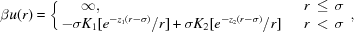where σ is the hard core diameter (and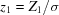,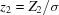), can be shown to be a very versatile model for the study of the structural properties of a colloidal system. In fact, the potential above can have the form of a short-range attraction followed by a long-range repulsive tail, when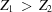, in which case it can be applied to globular proteins (Lawlor et al., 2004; Yu et al., 2005; Liu et al., 2005b), fullerenes at high temperatures (Guérin, 1998) and possibly charged colloid-polymer mixtures. It can also take on the form of a short-range repulsive shoulder followed by a longer range attractive tail, when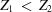, in which case it may describe the effective interaction of soft' attractive particles in solution, like normal micelles in water and reverse micelles in oil. The Ornstein–Zernike (OZ) equation for the direct correlation function c(r) for the 2Y fluid can be solved either analytically with mean spherical approximation (MSA) (Høye & Blum, 1977; Blum & Høye, 1978) or numerically with some suitable closure relation. The calculation method devised by Liu et al. (2005a), which provides the complete set of roots for the relevant nonlinear equations, computes the static structure factor rapidly and efficiently and can be implemented for the purpose of fitting SANS intensity distributions. (The MATLAB code for the calculation of the structure factor is freely available from the authors.)

The comprehension of the rich phase behavior of proteins is still an unsolved problem, where even the celebrated Derjaguin–Landau–Verwey–Overbeek (DLVO) potential has shown some inadequateness and alternative models have been proposed (Tardieu et al., 1999; Lin et al., 2002; Liu et al., 2005a; Liu et al., 2005b). A striking feature recently found with SANS and small-angle X-ray spectra from lysozyme protein solutions has been the appearance of a low-Q peak in addition to the first diffraction peak, which implies the formation of equilibrium clusters (Stradner et al., 2004). This phenomenon is believed to be due to the competing effect of a strong short-range attraction and a weak screened Coulomb repulsion. For an appropriate relative ratio of attraction to repulsion strength, this feature can be qualitatively reproduced by a 2Y model fluid (Broccio et al., 2006).

The 2Y model potential, compared with the very popular 1Y model of Hayter & Penfold (1981), is more flexible so that it is able to simulate, with an appropriate choice of parameters, a wide variety of realistic potentials, such as DLVO-like or Lennard–Jones-like potentials. We shall show in this paper that the model is able to reproduce the measured SANS intensity distributions in two specific cases, namely charged protein solutions, having a short-range attraction plus a repulsion, and triblock copolymer micellar solutions, simulating their soft-core repulsion plus a weak attraction.

### 2. The 2Y model for the structure factor

The static structure factor of a simple liquid is usually obtained by solving the OZ equation for the direct correlation function c(r)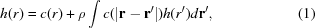where h(r) is the total correlation function, accompanied by a suitable closure relation. Exploiting the theoretical method given by Høye & Blum (1977), the recent numerical method developed by Liu et al. can calculate the analytical structure factor with MSA closure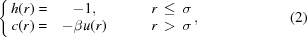in the case of a two-Yukawa interaction potential, obtaining an expression for c(r) inside the hard core. After some algebraic calculations, in the case of a 2Y potential, one is left with the following expression for its Fourier transform c(Q) (Q being the modulus of the exchanged wavevector):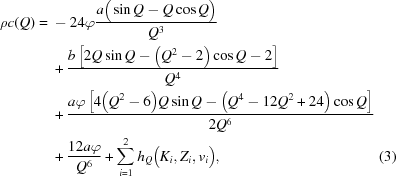where φ is the volume fraction and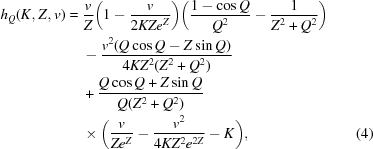whose coefficients {a, b, vi} (i = 1, 2) are calculated in terms of the known parameters {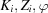} (i = 1, 2), with automatic criteria of good root selection, are as explained in detail in Liu et al. (2005a).

The static structure factor S(Q), which can be extracted by SANS experiments, can be therefore calculated through the usual relation in Fourier space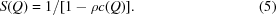The OZ equation can be also solved numerically with the hypernetted-chain (HNC) closure. In the presence of a strong short-range attraction, the HNC method shows better accuracy than the MSA method (Broccio et al., 2006). A plot of the structure factor obtained solving the OZ equation numerically with HNC (Springer et al., 1973) for a 2Y fluid having a strong short-range attraction and a weak long-range repulsion (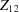), at four different volume fractions, 10%, 15%, 20% and 25%, is shown in the upper panel of Fig. 1. In the lower panel of the figure, the theoretical structure factors at three of the volume fractions above (10%, 15%, 20%) are compared with results of Monte Carlo (MC) simulations performed on 800 particles in a cubic box with periodic boundary conditions. The potential parameters used in the simulation are the same of those coming from a typical HNC fitting of SANS data from cytochrome c proteins in solution, that will be shown in §5. The qualitative agreement with simulation data for these sets of parameters is satisfactory, being also the low-Q peak closely reproduced by the theory. Such a peak, signature of a cluster–cluster correlation (Sciortino et al., 2004), does not shift upon changing volume fraction, demonstrating the invariance of the inter-cluster interaction in a wide range of volume fractions, in agreement with the findings of Stradner et al. (2004).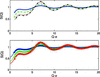Figure 1 (Top panel) theoretical HNC structure factors of a 2Y fluid interacting with short-range attraction and long-range repulsion, using the same potential parameters of the HNC fitting of cytochrome c data, at volume fractions 10% (blue solid line), 15% (green dashed line), 20% (red dotted line) and 25% (black dash-dotted line). (Bottom panel) same structure factors at volume fractions 10% (blue solid line), 15% (green solid line) and 20% (red solid line), compared with MC simulation results at volume fractions 10% (blue circles), 15% (green squares) and 20% (red diamonds).

We have also compared the radial distribution function g(r) of 2Y fluid computed analytically with MSA closure with the g(r) calculated directly during MC simulations in the case of a soft-core repulsion followed by a longer range attraction, that is when the 2Y potential has. Fig. 2shows such comparison at four different volume fractions, 35%, 40%, 45% and 50%. The good agreement of 2Y model MSA predictions with the simulation data witnesses the accuracy of the MSA analytical solution also for this case.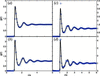Figure 2 Theoretical radial distribution functions g(r) (solid lines) for a 2Y fluid interacting with a soft-core repulsion and a longer range attraction, using typical potential parameters from the fitting of micellar solutions data, at the following volume fractions: (upper left) 35%; (lower left) 40%; (upper right) 45%; (lower right) 50%. Blue circles represent MC simulation data.

### 3. Materials

The protein cytochrome c, extracted from horse heart, is made of 104 amino acids and has a molecular weight of 12384 with dimensions a × b × b = 15 × 17 × 17 Å3. It was purchased from Sigma Chemical Company and dissolved in deuterated water purchased from Cambridge Isotopes. The isoelectric point was pI = 10.2, so that protein charge can be varied by tuning the pH of the solution. Samples were dialyzed three times and the final pD was 9.5. A few days were allowed between preparation and experiments, in order to let hydrogen and deuterium atoms to exchange. The pD was checked with an ISFET pH meter before and after the experiments, and found to be stable within ±0.1 units. The triblock copolymer Pluronic L44, having a chemical formula (EO11-PO21-EO11) and an average molecular weight of 2200, was given as a gift from the BASF Corporation. It belongs to a family of polymer surfactants widely used in industry for adhesion, detergency, wetting, foaming, emulsification, lubrication and solubilization purposes. After a careful purification carried out to remove hydrophobic impurities, the copolymer was dissolved in deuterated water with 99.9% D2O, purchased from Cambridge Isotopes. The amount of single block and diblock impurities in solution may well be neglected at concentrations above a few percent, as shown by previous experimental studies (Liu et al., 1998).

### 4. Experimental

SANS experiments on cytochrome c have been performed using the 30 m small-angle scattering instrument at the NG7 station at NIST Center for Neutron Research (NCNR). The incident neutron wavelength, λ is 6 Å, with a spread Δλ /λ = 11% and two setups with different sample–detector distances have been used to cover the range of magnitude of exchanged wavevector, Q, from 0.004 Å−1 to 0.3 Å−1. SANS experiments on copolymer Pluronic L44 have also been performed at NG7 station in NCNR. A sample–detector distance 2 m is used to cover the Q range from 0.009 Å−1 to 0.3 Å−1. Scattering from moderately concentrated samples, ranging from 30% to 50% w/w, was measured in the temperature range 293 K to 338 K. Samples were prepared a week in advance with respect to the experiment and kept refrigerated, a condition under which copolymers are in the state of unimers.

### 5. Globular protein solutions: data analysis and discussion

The coherent scattering intensity (in cm−1) from a protein solution may be written as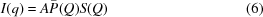where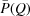is the normalized intraparticle structure factor (form factor) and S(Q) the interparticle structure factor and Q is the exchanged wavevector.

In the case of cytochrome c protein solution, the contrast A is given by Wu & Chen (1987)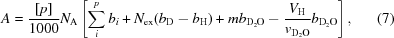where [p] is the molar concentration (mM) of the protein, NA = 6.022 × 1023 (Avogadro's number), bi the total scattering length of a cytochrome c molecule, Nex = 165 is the number of H/D exchange of the labile protons, m = 112 the number of hydrated water molecules, vH = (4π/3)ab2 the volume of a hydrated cytochrome c molecule and vD2O = 30 Å3 the volume of a water molecule.

The structure factor S(Q) is computed using both the MSA analytical expression described above and the numerical HNC method (Broccio et al., 2006). Accounting for the slight ellipticity of the molecule, the 2Y model structure factor has to be corrected with the decoupling approximation (Chen, 1986) as follows,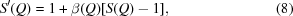to provide an average over orientation, where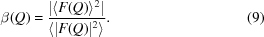The experimental intensity distributions are plotted, along with the fittings, for two different concentrations in Fig. 3, which clearly show a good agreement between the 2Y model predictions and the SANS data. During fitting, we have required that the parameters of the short-range attraction (K1, Z1), charge number and protein radius should be the same for both concentrations. The top panels show the fitting results obtained by the MSA method, while the bottom ones show the fitting results obtained by the HNC method. The volume fractions obtained are 10.5% and 14.2% for the MSA method, and 10.0% and 14.9% for the HNC method. Other fitting parameters are given in Table 1.

 Table 1Results for fitting parameters of SANS data from cytochrome c protein solutions at two different concentrations
Fitting method K1 Z1 Charge number a (Å)
MSA 2.8 18 1.5 14.7
HNC 1.6 14 1.7 14.7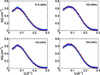Figure 3 Comparison of the MSA (top panels) and HNC (bottom panels) fitting for two SANS spectra of cytochrome c protein solutions at 10% and 15% volume fraction. Symbols represent experimental data, lines are fits with 2Y model.

Both MSA and HNC fittings give similar results for the charge number and a, although HNC finds a smaller value for the strength of the short-range attraction, K1. Since MC simulation shows that HNC is generally more accurate, the short-range attraction of cytochrome c protein in solution should be around 1.6 kBT at pH ∼9.5. As a comparison, we have fitted the SANS results for lysozyme solutions. Table 2lists the fitting parameters obtained from both the MSA method and HNC method. The results with the MSA method are obtained from Liu et al. (2005b).

 Table 2Results for fitting parameters of SANS data from lysozyme protein solutions at two different concentrations
Volume fraction Fitting method K1 Z1 Charge number a (Å)
10% MSA 6.9 14 7.0 21.1
HNC 4.3 14 7.1 21.1
20% MSA 7.7 15 6.8 21.2
HNC 3.8 15 6.0 21.0

Similar to the results obtained from cytochrome c protein solutions, both methods give similar values for the lysozyme solutions for the charge number and a, and the HNC method shows a smaller value of K1. The strength of short-range attraction from the HNC method is about 4 kBT, which is in a good agreement with the results obtained at different volume fractions and ionic strength using the HNC method (Tardieu et al., 1999).

### 6. Copolymer micellar solutions: data analysis and discussion

It is well known that Pluronic triblock copolymer surfactants dissolved in water, owing to a higher hydrophobicity of the inner poly(oxypropylene) blocks with respect to the outer poly(oxyethylene) ones, are able to self-assemble into thermodynamically stable micelles. This happens at any given concentration when temperature is increased above some critical value. The onset of the disordered micellar phase is signalled experimentally by a strong increase (more than an order of magnitude) in the scattering intensity with respect to the case of the unimer phase. The determination of the critical micellar concentration (cmc) and the corresponding critical micellar temperature (cmt) curve is approximate, since there exists no sharp micellization boundary, but a quite broad region of coexistence of micelles and single copolymer chains. The data sets examined in this paper are located well above the cmc–cmt curve, in the disordered micellar region of the phase diagram, that can be treated by means of equilibrium liquid theory. The coherent scattering intensity (in cm−1) from L44 copolymer micelles in solution is written as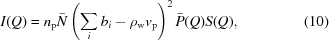where np is the number of polymer chains per unit volume (cm3),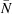is the average aggregation number of a micelle, bi the scattering length of a single copolymer molecule, vp the volume of a single copolymer and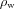the scattering length density of the solvent.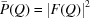is the normalized form factor and S(Q) the structure factor.

For the form factor, we assume a core–shell internal structure of the micelle, following the approach already used for this family of copolymers (Liu et al., 1998). Therefore the micelle consists of a dry hydrophobic core made only of PPO blocks, surrounded by a hydrated corona made of PEO blocks and solvent molecules. For a given micelle having core radius a and external radius R, the (normalized) function F(Q) may be written as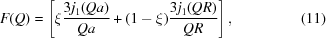where j1(Q) is the first-order spherical Bessel function and the dimensionless quantity ξ describes the scattering length distribution between core and corona. It is given as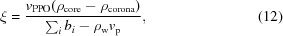where ρcore = (bPPO/vPPO) and ρcorona = (bPEO + HbD2O)/(vPEO + HvD2O), being vPPO and vPEO the volume of a PPO block and a PEO block respectively, bPPO and bPEO the scattering length of a PPO block and a PEO block, respectively. In turns, the hydration number H, defined as the average number of solvent molecules per copolymer chain, is determined through the condition of volume conservation in the micellar corona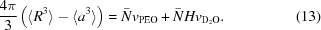Assuming, in accordance with the chemical information on the sample, that micelles are moderately polydisperse, the normalized form factor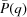can be defined in terms of an average over the size distribution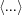, that is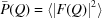. The average radius of the PPO core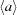is tied only to the average aggregation number through the condition of volume conservation in the core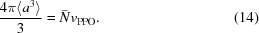The micelle volume fraction φ and average aggregation numbergive the average micelle radius through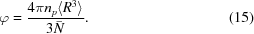In particular, we assume that the micelle radius R follows a Schultz size distribution, having mean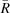and width ζ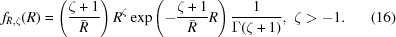The mean of the distributioncan be then derived from the corresponding average, since the j-th moment of the Schultz distribution is given by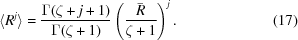The polydispersity index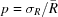, with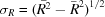root-mean-square deviation from the mean, is thus given by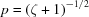. For obvious geometrical reasons, the core radius a follows the same kind of distribution, having the same width parameter ζ of the distribution of R, so that an analogous relation to equation (17)holds for a.

For the calculation of the structure factor, we adopt a 2Y interaction potential made of a short-range repulsive shoulder plus a longer range weak attractive tail, to describe the effective micelle–micelle interaction. We use the MSA analytical expression for S(Q), which has been proven to be accurate in Fig. 2by comparing to the MC simulation results. The 2Y model structure factor, in order to account for the effect of micelle size polydispersity (Kotlarchyk et al., 1984), has to be corrected in the following way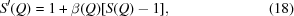with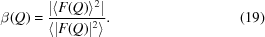Previous knowledge of micellar systems already suggested the presence of an effective attraction due to the hydrophobicity unbalance within the micelle, besides a purely hard-core repulsion. We add the assumption that the inside of a micelle is not completely bulky, substantiating the hypothesis of a `soft-core' type of interaction between micelles, acting on a range which is intermediate between the hard-core diameter and the attraction range. The hard-core diameter σ, in terms of which the interaction is computed, corresponds then to the portion of the micelle that is completely impenetrable by other micelles.

The intensity distributions from these copolymer micellar solutions in the range of weight concentration between 30% and 50%, have been successfully analyzed in the temperature range from 293 K to 338 K using the 2Y model. A gradient-searching nonlinear least-squares fitting has been carried out, using the following fitting parameters: volume fraction φ, average aggregation number, polydispersity index ζ, potential parameters repulsion strength K2 and attraction strength K1. The polydispersity index ζ do not show a clear dependence on temperature and concentration, and stays always centered at a value corresponding to a ∼30% size spread. The potential range parameters Z1 and Z2 (being Z1 < Z2), defining the shape of the model potential, were kept fixed during the fitting process. The range of the short-range repulsive Yukawa shoulder is Z2 ∼ 28, whereas that of the attractive Yukawa tail is Z1 ∼ 13, to describe the effective micelle–micelle interaction.

Fig. 4shows in four panels the SANS data and corresponding fitting results for two different concentrations of L44 copolymer micellar solutions at two temperatures. The values obtained for the fitting parameters are listed in Table 3.

 Table 3Results for fitting parameters of SANS data from copolymer micellar solutions at different concentrations and temperatures
Concentration (%) Temperature (K) φζ K1 K2
30 317 0.320 65 10.02 1.53 6.85
30 321 0.320 78 8.18 1.62 7.89
40 317 0.420 65 10.39 1.53 6.67
40 321 0.420 70 10.18 1.63 6.97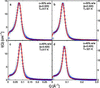Figure 4 SANS data from L44 copolymer micellar solutions and corresponding fitting curves at two different concentrations at two temperatures. Symbols represent experimental data, lines are fits with 2Y model.

The volume fraction obtained from fitting is uniquely related to the polymer weight concentration and its value is indeed stable with temperature. The average micelle diameter obtained from fitting, just above the cmt, is ∼80 Å, while its hydrophobic core size is ∼60 Å, both numbers depending on the aggregation number. The obtained value forincreases upon increasing temperature, due to the growth of micelles, as it is also witnessed by a shift towards lower Q of the first diffraction peak in the data. Looking at the change ofwith temperature, it seems that the micelle growth rate is steeper at lower volume fractions than it is at higher ones. The 2Y potential strength parameters K1 and K2 obtained from the fitting always support the picture of a steep repulsive shoulder besides the hard-core barrier. As an example, Fig. 5shows the 2Y potential (solid line) with the same parameters as used in the fitting of 40% micelle solution at 331 K, K1 = 4.71, Z1 = 13, K2 = 12.00, Z2 = 28. The increase of the attraction strength with temperature indicates that the fraction of micellar corona penetrable by other micelles enlarges, in agreement with the fact that the solvency of outer PEO parts decreases.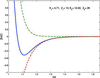Figure 5 Effective 2Y interaction potential (solid line) of copolymer micelles in solution, using the same parameters as those coming from fitting of SANS data of 40% solution at 331 K. The green dash-dotted line is the repulsive contribution, while the red dashed line is the attractive contribution.

### Acknowledgements

The authors acknowledge a support grant from the Materials Science Division of US DOE, DE-FG02-90ER45429, and the benefit from the affiliation to the EU Marie Curie Research and Training Network on Dynamical Arrest of Soft Matter and Colloids (Contract No. MRTN-CT-2003-504712).

### ReferencesJOURNAL OFAPPLIEDCRYSTALLOGRAPHY